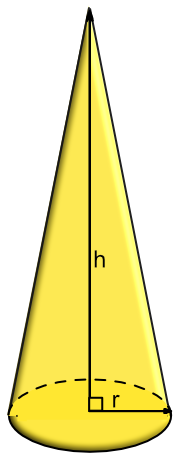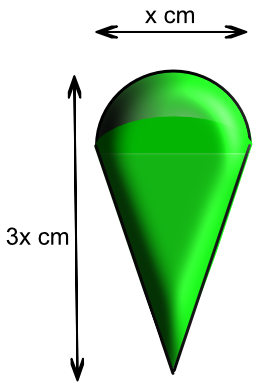Volume of a Cone

## Volume of a Cone

The volume of a cone is:

V=pir^2frac(h)(3)NOTE that h is the vertical height of the cone.

A way of remembering this is frac(1)(3) xx text(vertical height ) xx text( base area)

## Example 1

A cone has a vertical height of 12cm and a radius of 5 cm. What is the volume of the cone, to 3 significant figures?

 For a cone: Volume = pir^2frac(h)(3 Substitute = pi(5)^2 xx frac(12)(3) =314

## Example 2

The shape shown below consists of a hemisphere placed on a cone. The total volume of the shape is 25 cm3. The overall width of the shape is x cm, and the total vertical height of the shape is 3x cm.

What is the value of x? Give your answer to 1 decimal place.The radius of the hemisphere is frac(x)(2).

The vertical height of the cone is the height of the shape minus the radius, or 3x - frac(x)(2)

 Hemisphere: Volume = frac(1)(2) xx frac(4)(3)pir^3 V_h = frac(1)(6) xx frac(4)(3)pifrac(x)(2)^3 = frac(1)(12)pix^3 Cone: Volume = pir^2h V_c = pifrac(x)(2)^2(3x - frac(x)(2)) = pifrac(x)(2)^2(frac(6)(2)x - frac(x)(2)) = pifrac(5)(8)x^3 Total = V_h + V_c 25 = frac(1)(12)pix^3 + pifrac(5)(8)x^3 25 = frac(17)(24)pix^3 110.8797 = x^3 4.804 = x